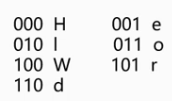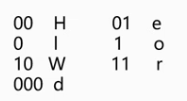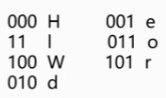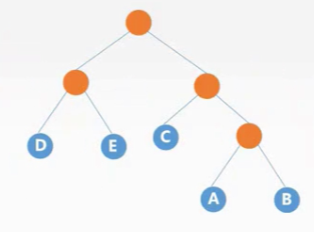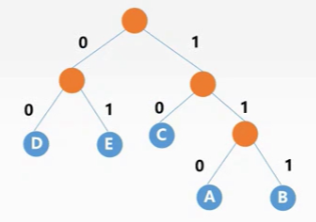# 第四章：树与二叉树（树与二叉树的应用：二叉排序树、二叉平衡树、哈夫曼树）

## 1.二叉排序树

• 若左子树非空,则左子树上所有结点关键字值均小于根结点的关键字
• 若右子树非空,则右子树上所有结点关键字值均大于根结点的关键字
• 左、右子树本身也分别是一棵二叉排序树。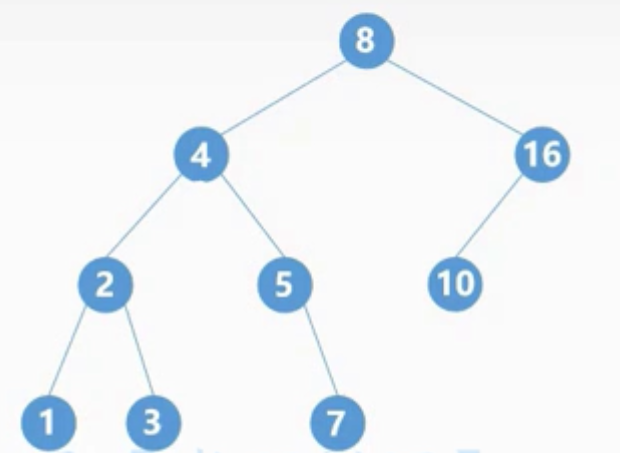### 1.1二叉排序树的查找

• 二叉树非空时,查找根结点,若相等则查找成功;
• 若不等,则当小于根结点值时,查找左子树;当大于根结点的值时,查找右子树。
• 当查找到叶节点仍没查找到相应的值,则查找失败。BSTNode *BST_Search(BiTree T,ElemType key,BSTNode *&p){
p = NULL; //双亲结点置为空(根结点没有双亲结点)
while(T != NULL && key != T->data){//树非空且关键字不匹配
p = T; //p指向改结点
if(key < T->data){
T = T->lchild; //循环查找左子树
}else{
T = T->rchild; //循环查找右子树
}
}
return T;
}


### 1.2二叉排序树的插入

• 若二叉排序树为空,则直接插入结点;
• 若二叉排序树非空,当值小于根结点时,插入左子树;当值大于根结点时,插入右子树;当值等于根结点时不进行插入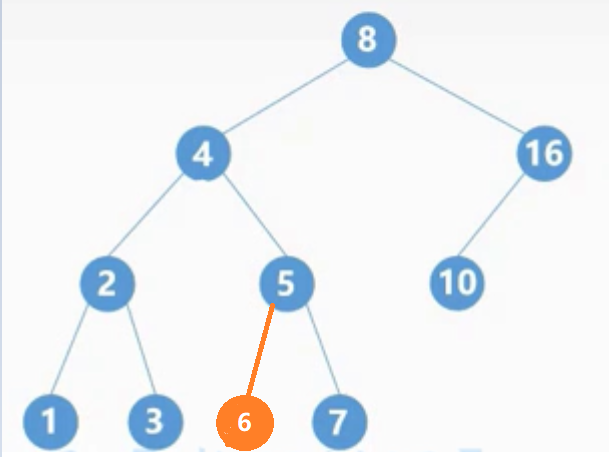//参1 二叉树(注意是引用)，参2 插入值
int BST_Insert(BiTree &T,KeyType k){
if(T==NULL){ //树为空
T = (BiTree)malloc(sizeof(BSTNode));
T->key = k;
T->lchild = T->rchild = NULL;
return 1;
}else if(k == T->key){ //相同不插入
return 0;
}else if(k < T->key){//小于插入左子树中，递归调用
return BST_Insert(T->lchild,k);
}else{//大于插入右子树中，递归调用
return BST_Insert(T->rchild,k);
}
}


### 1.3构造二叉排序树

//参3 插入结点的数量
void Create_BST(BiTree &T,KeyType str[],int n){
T = NULL;
int i=0;
while(i<n){
BST_Insert(T,str[i]);
i++;
}
}


### 1.4二叉排序树的删除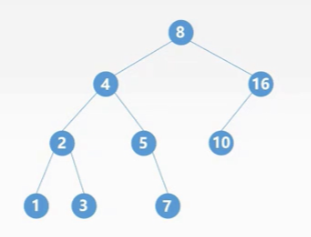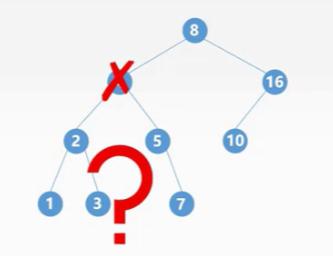• 1.若被删除的结点z是叶子结点则可以直接删除，不影响
• 2.如果被删除的结点z只有一个子树，则让z的子树成为z父结点的子树，带题z结点。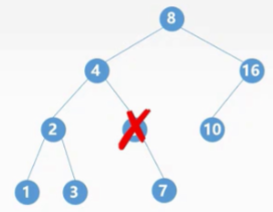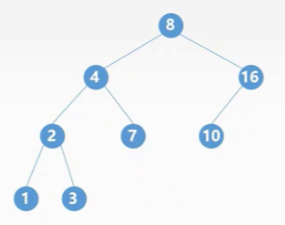• 3.若被删除的结点z有两颗子树，则让z的中序序列直接后继代替z，并删去直接后继结点。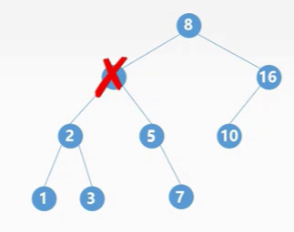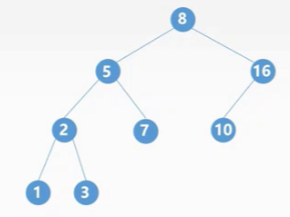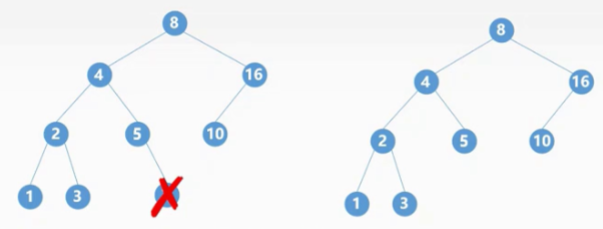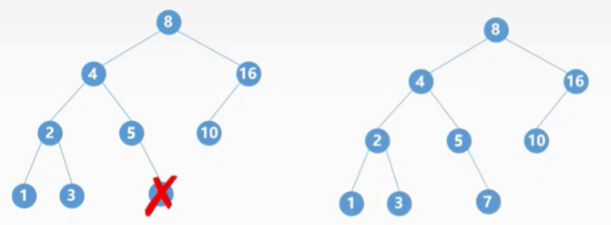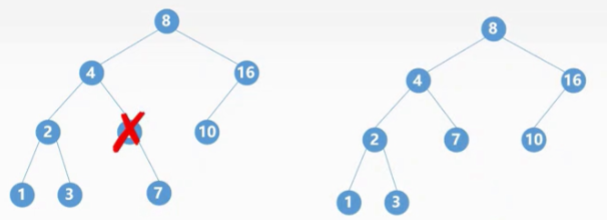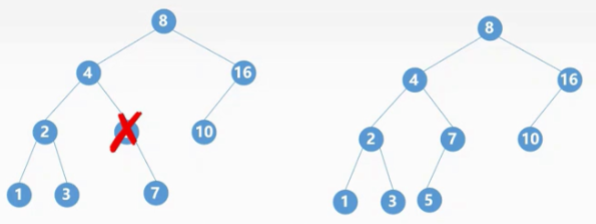### 1.5查找效率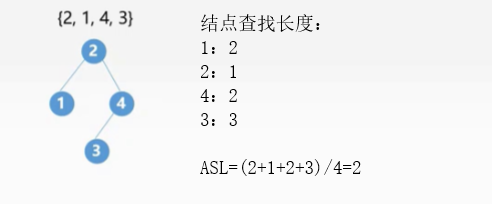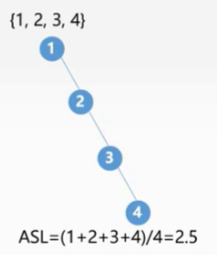## 2.平衡二叉树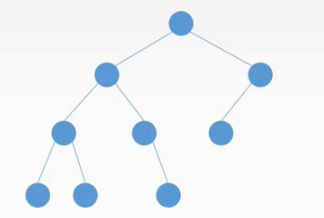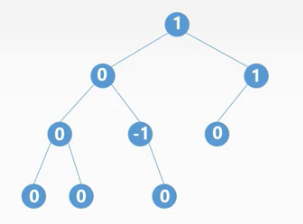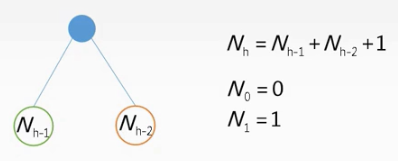N3=N2+N1+1; =》N2=N1+N0+1=2; =》N3=2+1+1=4;

### 2.1平衡二叉树的判断

• 判断左子树是一棵平衡二叉树
• 判断右子树是一棵平衡二叉树
• 判断以该结点为根的二叉树为平衡二叉树
判断条件
若左子树和右子树均为平衡二叉树且左子树与右子树高度差的绝对值小于等于1,则平衡。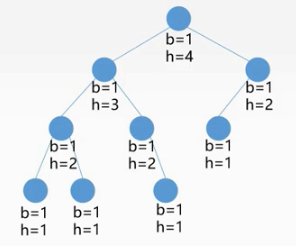//参1 该棵树的根节点
void Judge_AVL(BiTree bt,int &balance,int &h){
//左子树平衡性左子树高度 右子树平衡性右子树高度
int bl=0,br=0,hl=0,hr=0;
if(bt==NULL){//如果根节点为空
h=0; //高度设为0
balance=1; //并且是平衡的
}else if(bt->lchild==NULL&&bt->rchild==NULL){
//左子树和右子树都为空
h=1; //高度为1
balance=1; //平衡的
}else{
Judge_AVL(bt->lchild,bl,hl); //判断左子树
Judge_AVL(bt->rchild,br,hr); //判断右子树
//下面计算该节点为根二叉树的高度
//首先判断哪个子树的高度高，然后加1即可
if(hl>hr){
h=hl+1;
}else{
h=hr+1;
}
//判断平衡性
// abs 是取绝对值
if(abs(hl-hr)<2&&bl==1&&br==1){
balance=1;
}else{
balance=0;
}
}
}


## 3.平衡二叉树的插入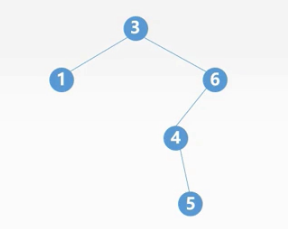### 3.1LL平衡旋转(右单旋转)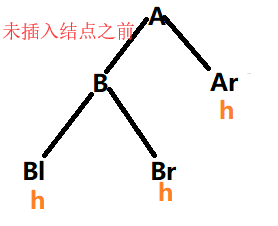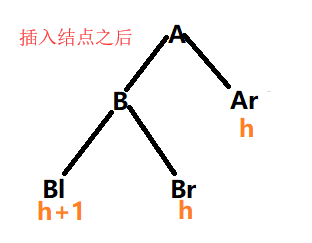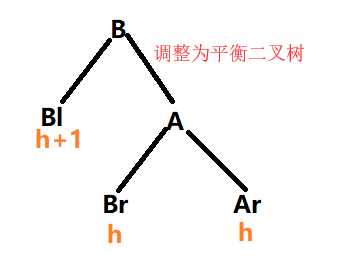### 3.2RR平衡旋转(左单旋转)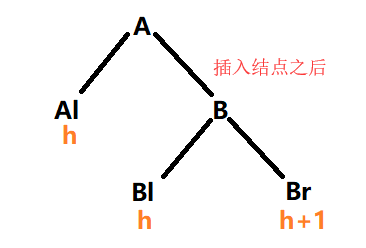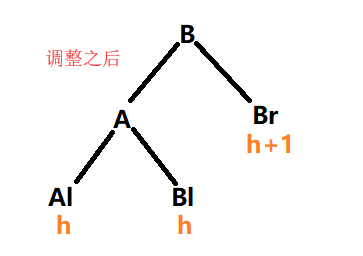### 3.3LR平衡旋转(先左后右双旋转)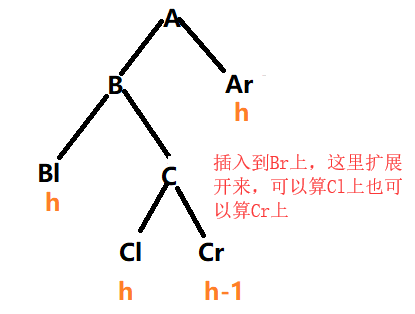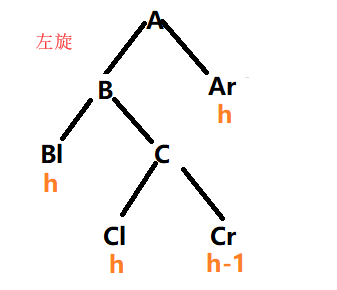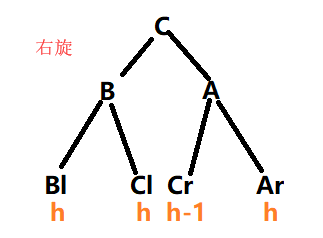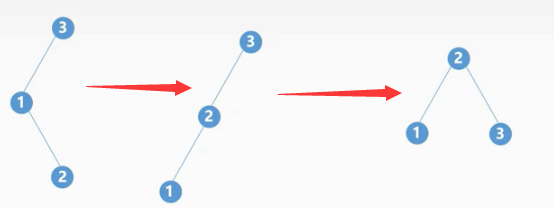### 3.4RL平衡旋转(先右后左双旋转)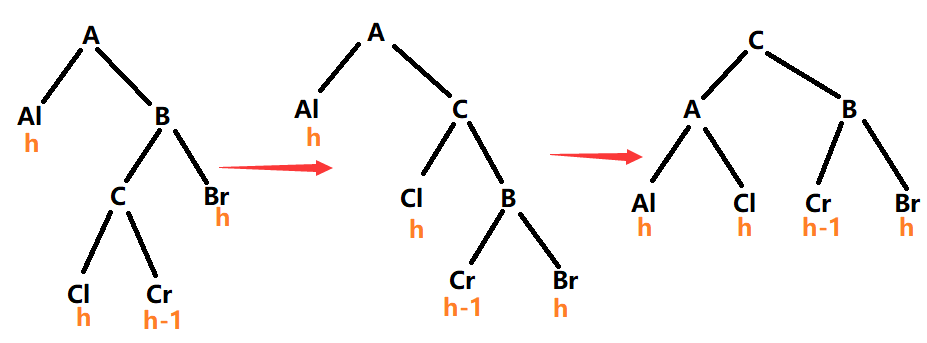## 4.带权路径长度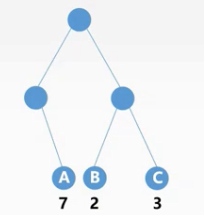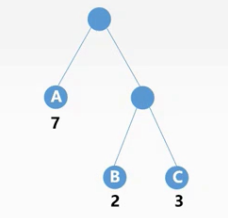## 5.哈夫曼树

### 5.1哈夫曼树的构造

• 将n个结点作为n棵仅含有一个根结点的二叉树,构成森林F
• 生成一个新结点,并从F中找出根结点权值最小的两棵树作为它的左右子树,且新结点的权值为两棵子树根结点的权值之和
• 从F中删除这两个树,并将新生成的树加入到F中
• 重复2,3步骤,直到F中只有一棵树为止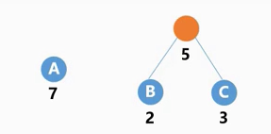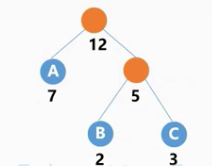### 5.2哈夫曼树的性质

• 每个初始结点都会成为叶节点,双支结点都为新生成的结点
• 权值越大离根结点越近,反之权值越小离根结点越远
• 哈夫曼树中没有结点的度为1
• n个叶子结点的哈夫曼树的结点总数为2n-1,其中度为2的结点数为n-1

### 5.3哈夫曼编码